## Filter Combinator

Post your ideas and suggestions how to improve the game.
Trebor
Fast Inserter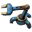Posts: 179
Joined: Sun Apr 30, 2017 1:39 pm
Contact:

### Filter Combinator

##### Filter Combinator
A combinator that uses the red and green networks as separate inputs to an operation to produce separate output values for the red and green networks.

##### What?
I propose a new filter combinator. This combinator would take the red and green networks as separate inputs (not added together) and an operation and produce separate red and green outputs. Each operation would have the green network act on the red network to produce red output and have the red act on the green to produce green output. For example the subtract operation would subtract green from red to produce new red values and subtract red from green to produce new green values.

Each operation would use one of two methods to produce its output, the normal mode (N) would be to use the current value if the other network’s was zero otherwise apply the operation. In filter mode (F) the default value is zero if the other network’s was zero otherwise apply the operation. Filter mode is used for any operation if using normal mode would produce the same output values on both networks.

Operations:
Subtract (N)- subtract one network from the other.
Multiply (N)- multiply non-zero values on one network by the other
Divide (N)- divide non-zero values on one network from the other.
Minimum (N)- a non-zero value on the other network that is less than the value on the current network is used otherwise the value from current network is used. A negative value on the other network may be used as the minimum but a zero value can’t (the value of the current network would be used).
Maximum (N)- maximum non-zero value is used. A zero value on the current network is larger than a negative value on the other but a zero on the other network would be ignored (the negative on the current network would be used).
Exchange (N)- use non-zero values from the other network, when the other network has a zero value the value on the current network is used.
Bit ops (and/or/xor) (N)- performs operation when other network is non-zero otherwise retains value from current network.
Filter (F)- non-zero values on one network would pass the value of the matching symbols of other network. Example: a non-zero green iron ore would pass the red iron ore value but a zero green copper ore would produce zero red copper ore.
Addition (F)- Add the values of the two networks together but if the other network’s value is zero produce a zero value.

All operations would take one tick.

Suggest a separate blue science technology.

Recipe contains red circuit and/or other combinators.

Combinator size should be 1x2.

##### Why?
While all of these operations can be done with the current combinators to do so takes many combinators (lots of space) is error prone and can not be done in one tick.

IGotBaconSoda
Burner Inserter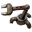Posts: 15
Joined: Thu Mar 24, 2016 7:45 pm
Contact:

### Re: Filter Combinator

+1, great idea and something I could use to improve a few designs (and invent more)

Makes the circuit network a bit more intuitive to be able to weigh each wire as well; making 0 and 1 signals relevant if red and green both carry 0 or 1 high, for example.

ikarikeiji
Long Handed Inserter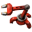Posts: 92
Joined: Sun Jul 12, 2015 6:28 pm
Contact:

### Re: Filter Combinator

Can you give practical use cases for each of the filter operations you describe? These sound rather arbitrary so far.

Pi-C
Fast InserterPosts: 167
Joined: Sun Oct 14, 2018 8:13 am
Contact:

### Re: Filter Combinator

ikarikeiji wrote:
Tue Jan 08, 2019 1:25 pm
Can you give practical use cases for each of the filter operations you describe? These sound rather arbitrary so far.
I think this thread shows a valid example: Use the value of a signal on the red wire only if that signal (arbitrary value) is also sent over the green wire, discarding the value from the green signal. I needed that for a train dispatcher once, although I don't quite remember the details now.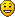A good mod deserves a good changelog. Here's a tutorial (WIP) about Factorio's way too strict changelog syntax!

Sad_Brother
Fast InserterPosts: 117
Joined: Mon Jan 08, 2018 4:54 pm
Contact:

### Re: Filter Combinator

Trebor wrote:
Fri Aug 17, 2018 1:41 pm
Operations:
Subtract (N)- subtract one network from the other. - How many I need above I have?
Multiply (N)- multiply non-zero values on one network by the other - I have a cost of each item, How much they cost all?
Divide (N)- divide non-zero values on one network from the other. - How many precents of needed supply I have?
Minimum (N)- a non-zero value on the other network that is less than the value on the current network is used otherwise the value from current network is used. A negative value on the other network may be used as the minimum but a zero value can’t (the value of the current network would be used). - Why should I need it?
Maximum (N)- maximum non-zero value is used. A zero value on the current network is larger than a negative value on the other but a zero on the other network would be ignored (the negative on the current network would be used). - Why should I need it?
Exchange (N)- use non-zero values from the other network, when the other network has a zero value the value on the current network is used. - Why should I need it?
Bit ops (and/or/xor) (N)- performs operation when other network is non-zero otherwise retains value from current network. - Why should I need it? Extremely cyphered codes?
Filter (F)- non-zero values on one network would pass the value of the matching symbols of other network. Example: a non-zero green iron ore would pass the red iron ore value but a zero green copper ore would produce zero red copper ore. - Can use Multiply by 1 from above to exclude unneeded signals.
Addition (F)- Add the values of the two networks together but if the other network’s value is zero produce a zero value. - Why should I need it?

jamiechi1
Inserter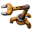Posts: 26
Joined: Wed Jan 03, 2018 10:12 pm

### Re: Filter Combinator

I agree. I really dislike the implied 'wired-or' functionality with combinators. Also having built in logic gates would be good. I have been designing logic circuits for years, and find combinators in their current form very difficult to master.

Trebor
Fast InserterPosts: 179
Joined: Sun Apr 30, 2017 1:39 pm
Contact:

### Re: Filter Combinator

Here is another use case for this:
viewtopic.php?f=214&t=64775&p=397462#p397462
Optera wrote:
Thu Feb 07, 2019 7:39 am
To prevent the above I like to abuse factorio's divisions truncation to always get multiples of train capacity. This takes 2 arithmetic combinators for every item.
Barrels=(Barrels/barrel_capacity) * barrel_capacity
Slag=(Slag/slag_capacity) * slag_capacity
If number of item were on red and capacity were on green it would only take one combinator regardless of how many items you wanted to track.

Optera
Smart Inserter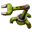Posts: 2035
Joined: Sat Jun 11, 2016 6:41 am
Contact:

### Re: Filter Combinator

Trebor wrote:
Fri Feb 08, 2019 4:29 am
Here is another use case for this:
viewtopic.php?f=214&t=64775&p=397462#p397462
Optera wrote:
Thu Feb 07, 2019 7:39 am
To prevent the above I like to abuse factorio's divisions truncation to always get multiples of train capacity. This takes 2 arithmetic combinators for every item.
Barrels=(Barrels/barrel_capacity) * barrel_capacity
Slag=(Slag/slag_capacity) * slag_capacity
If number of item were on red and capacity were on green it would only take one combinator regardless of how many items you wanted to track.
Nope, this use case requires 2 combinators per item.

Trebor
Fast InserterPosts: 179
Joined: Sun Apr 30, 2017 1:39 pm
Contact:

### Re: Filter Combinator

Optera wrote:
Fri Feb 08, 2019 1:54 pm
Nope, this use case requires 2 combinators per item.
I should have said: only one of the filter combiners proposed in this thread.

Edit: Two total. I really shouldn’t do math late at night.

### Who is online

Users browsing this forum: SkiCarver